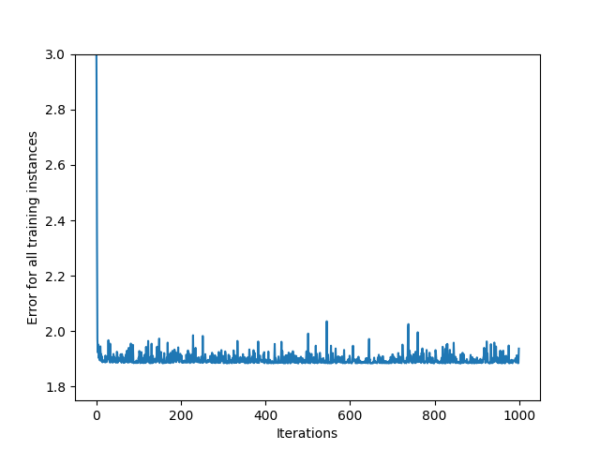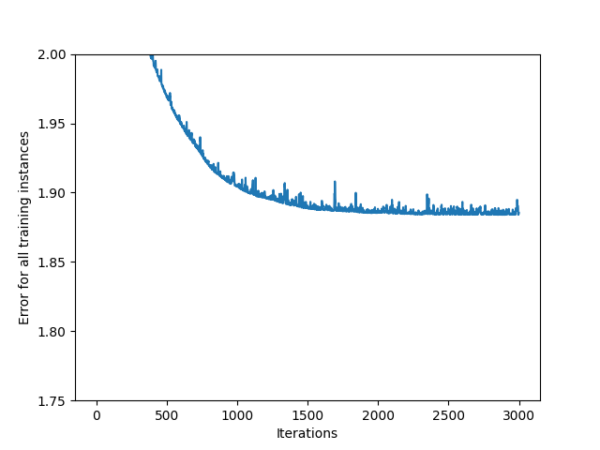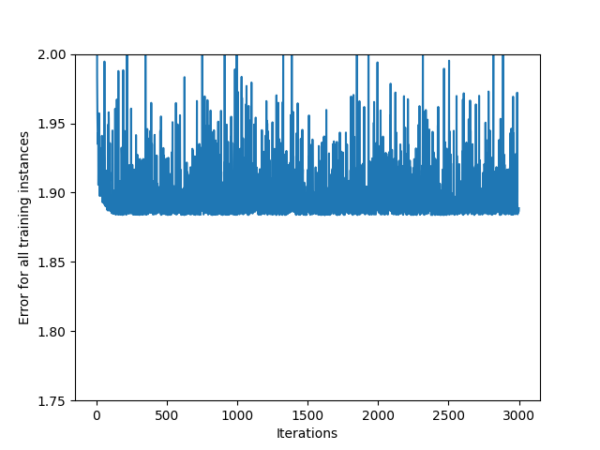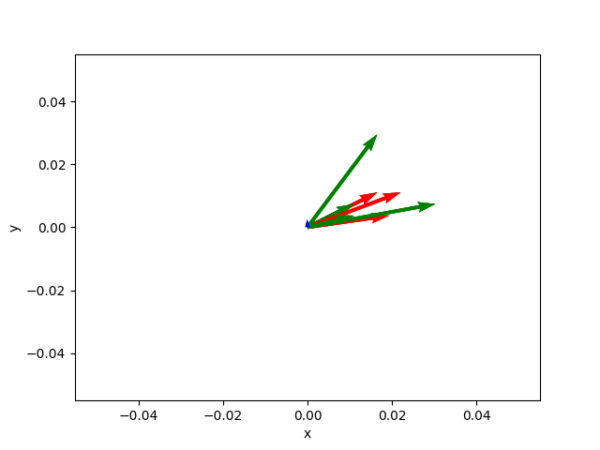# A Gentle, Minimalist intro to Machine Learning

Published Tagged
ML is taking the world over by storm in 2023. Here is an easy-to follow tutorial in pure python that explains the basics.

Hello everybody! Recently, I’ve been spending non-trivial amounts of time on the fascinating subject of artificial intelligence. It’s come a long way! With the release of Midjourney and ChatGPT, among other products, 2023 looks to be extremely promising, even revolutionary.

I’d like to recommend the following tutorial: https://realpython.com/python-ai-neural-network/

It is simple, sufficiently detailed, does not use tensor flow, and produces a picture at the end!

The complete code to run the example is reproduced below:

```import matplotlib.pyplot as plt
import numpy as np

class NeuralNetwork:
def __init__(self, learning_rate):
self.weights = np.array([np.random.randn(), np.random.randn()])
self.bias = np.random.randn()
self.learning_rate = learning_rate

def _sigmoid(self, x):
return 1 / (1 + np.exp(-x))

def _sigmoid_deriv(self, x):
return self._sigmoid(x) * (1 - self._sigmoid(x))

def predict(self, input_vector):
layer_1 = np.dot(input_vector, self.weights) + self.bias
layer_2 = self._sigmoid(layer_1)
prediction = layer_2
return prediction

layer_1 = np.dot(input_vector, self.weights) + self.bias
layer_2 = self._sigmoid(layer_1)
prediction = layer_2

derror_dprediction = 2 * (prediction - target)
dprediction_dlayer1 = self._sigmoid_deriv(layer_1)
dlayer1_dbias = 1
dlayer1_dweights = (0 * self.weights) + (1 * input_vector)

derror_dbias = (
derror_dprediction * dprediction_dlayer1 * dlayer1_dbias
)
derror_dweights = (
derror_dprediction * dprediction_dlayer1 * dlayer1_dweights
)

return derror_dbias, derror_dweights

def _update_parameters(self, derror_dbias, derror_dweights):
self.bias = self.bias - (derror_dbias * self.learning_rate)
self.weights = self.weights - (
derror_dweights * self.learning_rate
)

def train(self, input_vectors, targets, iterations):
cumulative_errors = []
for current_iteration in range(iterations):
# Pick a data instance at random
random_data_index = np.random.randint(len(input_vectors))

input_vector = input_vectors[random_data_index]
target = targets[random_data_index]

# Compute the gradients and update the weights
input_vector, target
)

self._update_parameters(derror_dbias, derror_dweights)

# Measure the cumulative error for all the instances
if current_iteration % 100 == 0:
cumulative_error = 0
# Loop through all the instances to measure the error
for data_instance_index in range(len(input_vectors)):
data_point = input_vectors[data_instance_index]
target = targets[data_instance_index]

prediction = self.predict(data_point)
error = np.square(prediction - target)

cumulative_error = cumulative_error + error
cumulative_errors.append(cumulative_error)

return cumulative_errors

input_vectors = np.array(
[
[3, 1.5],
[2, 1],
[4, 1.5],
[3, 4],
[3.5, 0.5],
[2, 0.5],
[5.5, 1],
[1, 1],
]
)

targets = np.array([0, 1, 0, 1, 0, 1, 1, 0])

learning_rate = 0.01
neural_network = NeuralNetwork(learning_rate)

training_error = neural_network.train(input_vectors, targets, 1000)

plt.plot(training_error)
plt.xlabel("Iterations")
plt.ylabel("Error for all training instances")
plt.savefig("cumulative_error.png")

```

And I would like to add some commentary of my own to this great tutorial.

First, the author writes the resulting error after training doesn’t decrease because the dataset is tiny, only 8 data points:But of course, an astute student would note that by decreasing the learning rate, and increasing the number of learning iterations, we can slightly reduce the error. Or if not reduce the error, at least reduce the variance of the error. The following is the plot of error after decreasing learning rate by 10-fold, and increasing iterations by 3-fold:If we zoom in, the original error looks like this, where smaller is better:So you can see the effects of reducing learning rate on the error.

My second commentary is: what does all of this mean? Let’s plot the input data:Red are vectors that should be categorized as “0”, green are categorized as “1”. Blue is the vector representing the learned weights of the network (there are only two, so I plot them as x,y).

Humans are great at pattern recognition. Just looking at the plot, you can see that the best (if overfit) predictor for this data would be a vector pointing to the average of red arrows, and an activation function to specify the radius around the average point, to define the red cluster.

Of course, the advantage of a neural network is that it is capable of classifying (and performing other operations) on much more complex data, where plotting inputs would perhaps be impossible. Nevertheless, for an introductory tutorial, I believe plotting inputs and outputs, whenever possible, is a nice way of developing intuition about mathematical concepts.

In a next article, we’ll go into details about various types of ANN’s, and write some further implementation of concepts.## By Victor Pudeyev

A technical lead and business developer residing in Austin, TX. I specialize in systems built with ruby, javascript and solidity.